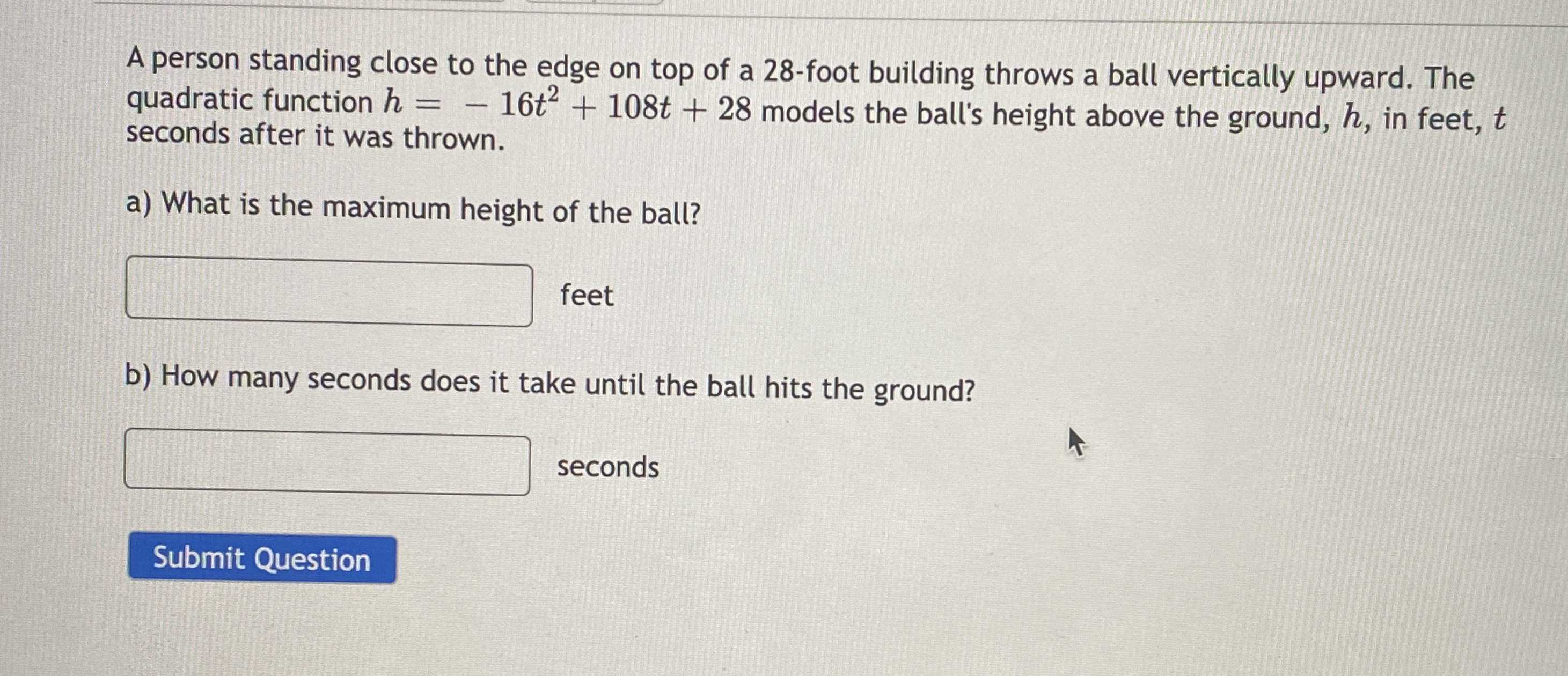### ¿Todavía tienes preguntas de matemáticas?

Pregunte a nuestros tutores expertos
Algebra
PreguntaA person standing close to the edge on top of a $$28$$ -foot building throws a ball vertically upward. The quadratic function $$h = - 16 t ^ { 2 } + 108 t + 28$$ models the ball's height above the ground, $$h$$ , in feet, $$t$$ seconds after it was thrown.

a) What is the maximum height of the ball?

b) How many seconds does it take until the ball hits the ground?